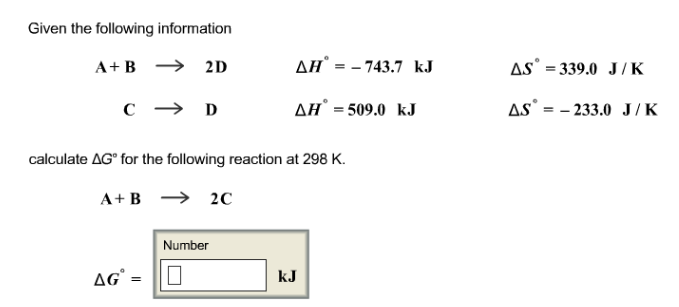# Problem: Given the following information A + B → 2D               ΔH° = -743.7 kJ               ΔS° = 339.0 J/K C → D                       ΔH ° = 509. 0 kJ              ΔS° = -233.0 J/K calculate ΔG° for the following reaction at 298 K.A + B → 2C

###### FREE Expert Solution
85% (140 ratings)###### Problem Details

Given the following information

A + B → 2D               ΔH° = -743.7 kJ               ΔS° = 339.0 J/K

C → D                       ΔH ° = 509. 0 kJ              ΔS° = -233.0 J/K

calculate ΔG° for the following reaction at 298 K.

A + B → 2C